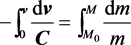﻿ 变质量运动火箭运动方程的推导

# 变质量运动火箭运动方程的推导Derivation of Motion Equation of Variable Mass Moving Rocket

Abstract: In this paper, the law of conservation of momentum is expressed: a system is free from external force or external force as zero or internal force is far greater than external force. The increment of the total momentum of the system is zero as the basis, and the equation of motion of fire and arrow with variable mass motion is obtained in a relatively concise way according to the momentum theorem. The derivation process in this paper will make it easier for readers to understand the equation of motion of variable mass.

1. 引言

$mv=\left(m+\text{d}m\right)\left(v+\text{d}v\right)+\left(-\text{d}m\right)\left(v-u\right)$ (1)

$mv=\left(m+\text{d}m\right)\left(v+\text{d}v\right)+\left(-\text{d}m\right)\left(v+\text{d}v-u\right)$ (2)

2. 变质量运动火箭运动方程的推导

$\left(m-\text{d}m\right)\text{d}v+C\text{d}m=0$ (3)

(3)式展开后可得：

$m\text{d}v-\text{d}m\text{d}v+C\text{d}m=0$ (4)

$m\text{d}v+C\text{d}m=0$ (5)

$-\frac{\text{d}v}{C}=\frac{\text{d}m}{m}$ (6)(7)

$v=C\mathrm{ln}\frac{{M}_{0}}{M}$ (8)

3. 结论

 沈鼎权. 火箭运动微分方程讲法的探讨[J]. 物理与工程, 1985(7): 40.

 张文富. 一个被忽视的问题[J]. 大学物理, 1983: 9.

 王永久. 关于物理教材中几个问题的讨论[J]. 工科物理教学, 1981: 3.

 史祥蓉. 火箭运动微分方程讲法的探讨[J]. 物理与工程, 2015(25): 3.

 吴松, 刘明柱, 高存功. 加强理科建设促进理工结合EJ [J]. 国家高级教育行政学院学报, 2001(4): 73.

 伯顿•R, 克拉克. 高等教育系统[M]. 王承绪, 译. 杭州: 杭州大学出版社, 1994.

 康颖. 大学物理[M]. 北京: 科学出版社, 2010.

 王建国. 关于变质量物体运动方程的讨论[J]. 河北北方学院学报(自然科学版), 2007(23): 3.

Top